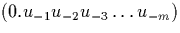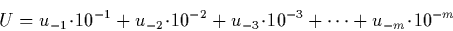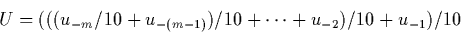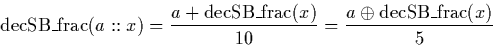Next: Signed Binary to Decimal Up: Decimal to Signed Binary Previous: Decimal integers to signed

### Decimal fractions to signed binary

Suppose we wish to compute the signed binary representation U of the decimal fractionusing signed binary operations, we simply evaluateTo compute this more efficiently, we observeWe can now use a simple recursive algorithm using the average and division by an integer operations which evaluates to zero on an empty input, but otherwise computes:Because we cannot represent decimal digits in signed binary streams, we replace a in the right hand side of the expression by a stream which represents a/24, and multiply the entire result by 24. Multiplication and division by powers of two can be performed easily using shift operations.

Martin Escardo
5/11/2000